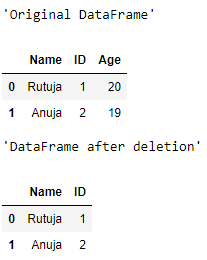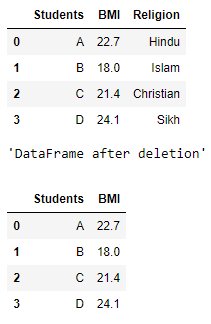# Delete a column from a Pandas DataFrame

Deletion is one of the primary operations when it comes to data analysis. Very often we see that a particular attribute in the data frame is not at all useful for us while working on a specific analysis, rather having it may lead to problems and unnecessary change in the prediction. For example, if we want to analyze the students’ BMI of a particular school, then there is no need to have the religion column/attribute for the students, so we prefer to delete the column. Let us now see the syntax of deleting a column from a dataframe.

Syntax:

```del df['column_name']
```

Let us now see few examples:

Example 1:

 `# importing the module ` `import` `pandas as pd ` ` `  `# creating a DataFrame ` `my_df ``=` `{``'Name'``: [``'Rutuja'``, ``'Anuja'``],  ` `         ``'ID'``: [``1``, ``2``], ``'Age'``: [``20``, ``19``]} ` `df ``=` `pd.DataFrame(my_df) ` `display(``"Original DataFrame"``) ` `display(df) ` ` `  `# deleting a column ` `del` `df[``'Age'``] ` ` `  `display(``"DataFrame after deletion"``) ` `display(df) `

Output :Note the column ‘Age” has been droped.

Example 2:

 `# importing the module ` `import` `pandas as pd ` ` `  `# creating a DataFrame ` `my_df ``=` `{``'Students'``: [``'A'``, ``'B'``, ``'C'``, ``'D'``],  ` `         ``'BMI'``: [``22.7``, ``18.0``, ``21.4``, ``24.1``],  ` `         ``'Religion'``: [``'Hindu'``, ``'Islam'``,  ` `                      ``'Christian'``, ``'Sikh'``]} ` `df ``=` `pd.DataFrame(my_df) ` `display(``"Original DataFrame"``) ` `display(df) ` ` `  `# deleting a column ` `del` `df[``'Religion'``] ` ` `  `display(``"DataFrame after deletion"``) ` `display(df) `

Output :Note that the unnecessary column, ‘Religion’ has been deleted successfully.

My Personal Notes arrow_drop_upCheck out this Author's contributed articles.

If you like GeeksforGeeks and would like to contribute, you can also write an article using contribute.geeksforgeeks.org or mail your article to contribute@geeksforgeeks.org. See your article appearing on the GeeksforGeeks main page and help other Geeks.

Please Improve this article if you find anything incorrect by clicking on the "Improve Article" button below.

Article Tags :

Be the First to upvote.

Please write to us at contribute@geeksforgeeks.org to report any issue with the above content.